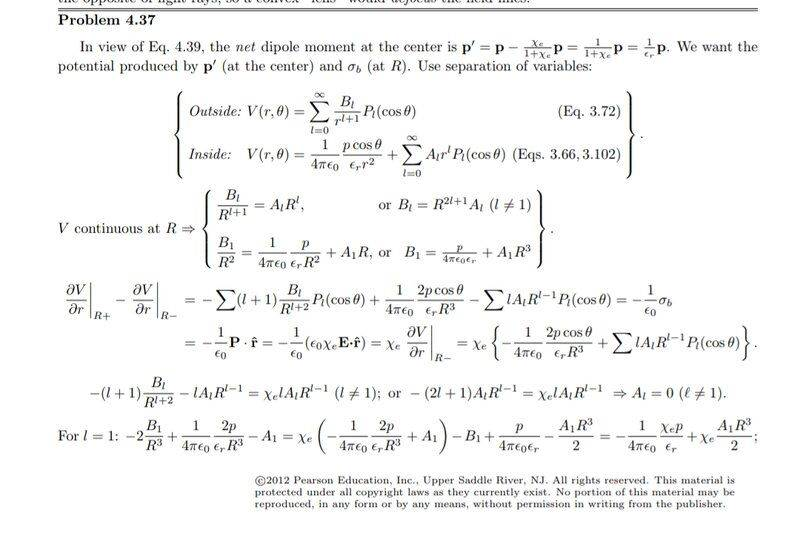# Laplace's equation when there is a point dipole

• Ahmed1029
In summary, the conversation discusses the use of Poisson's equation and Laplace's equation in solving for Laplace's equation when there is a point dipole within the volume. The solution involves treating the dipole as a boundary condition for the potential, which satisfies Laplace's equation for r>0. The conversation also talks about the contradiction that arises when using a point dipole in a problem, as it requires a singularity in the charge density at the location of the dipole. The conversation then goes into detail about the problem of a point charge and a dipole, and the resulting electrostatic potential and field.f

#### Ahmed1029

Homework Statement
A point dipole p is imbedded at the center of a sphere of linear
dielectric material (with radius R and dielectric constant r). Find the electric potential inside and outside the sphere.
Relevant Equations
Laplace's equation
I know the solution already, yet I don't understand why I'm able to solve laplace's equation when the volume has a point dipole within; I thought this required Poisson's eauation, which I have no experience in solving. Here is the relevant part of the solution•Delta2
Well Poisson's equation, $\nabla^2 \phi = \rho$, reduces to Laplace's equation ## \nabla^2 \phi = 0 ## at any point where the charge distribution is zero. Since the only charge in your problem iis at the origin, your potential satisfies Laplace's equation everywhere except at ##r=0##, which is what your posted solution is doing. Except your solution is also matching the small-r dependence with the potential of a dipole. You can think of it as treating the dipole as a boundary condition for the potential which satisfies Laplace's equation for ##r>0##.

Last edited:
••Ahmed1029, vanhees71 and Delta2
I think there is an inherent contradiction whenever we use a point dipole in a problem, because we say that the charge density ##\rho=0## everywhere, yet there is net dipole moment ##p\neq 0## where as ##p=\int\rho (\mathbf{r'}) (\mathbf{r-r'})d^3\mathbf{r'}## should be zero. If we say that the charge density isn't zero everywhere then we would have to solve Poisson's equation and not Laplace's equation.

•vanhees71
Of course, the charge distribution is not ##0## everywhere. It rather has a singularity at the location of the dipole.

It's well worth to consider this in some detail. So let's start from the somewhat simpler problem of point charge, which we put in the origin of our coordinate system. Also there ##\rho(\vec{r})=0## everywhere except at ##\vec{r}=0##, where the charge density must be singular. Formally that's written in terms of the Dirac distribution (where "distribution" here means "generalized function"), i.e.,
$$\rho(\vec{r})=q \delta^{(3)}(\vec{r}).$$
Now consider a dipole. Intuitively you can think about it as having two charges ##q## and ##-q##. Let's put the negative one at ##\vec{r}=0## and the positive one at some place ##\vec{d}##. Then the charge distribution is
$$\rho(\vec{r}) = q [\delta(\vec{r}-\vec{d})-\delta(\vec{r})]=-q \vec{d} \cdot \vec{\nabla} \delta(\vec{r}) + \mathcal{O}(q |\vec{d}|^2).$$
Now we consider the limit ##|\vec{d}| \rightarrow 0## such that ##\vec{p} =q \vec{d}=\text{const}##. Then the contributions to higher order vanish, and you get
$$\rho(\vec{r})=-\vec{p} \cdot \vec{\nabla} \delta^{(3)}(\vec{r}).$$
The electrostatic potential becomes
$$\Phi(\vec{r})=\int_{\mathbb{R}^3} \mathrm{d}^3 r' \frac{\rho(\vec{r}')}{4 \pi \epsilon_0 |\vec{r}-\vec{r}'|} =- \int_{\mathbb{R}^3} \frac{1}{4 \pi |\vec{r}-\vec{r}'|} \vec{p} \cdot \vec{\nabla}' \delta^{(3)}(\vec{r}').$$
Integration by parts gives [EDIT: corrected in view of #5]
$$\Phi(\vec{r})=+ \frac{1}{4 \pi \epsilon_0} \int_{\mathbb{R}^3} \mathrm{d}^3 r' \vec{p} \delta^{(3)}(\vec{r}') \cdot \vec{\nabla}' \frac{1}{|\vec{r}-\vec{r}'|} =\frac{\vec{p} \cdot \vec{r}}{4 \pi \epsilon_0 r^3},$$
i.e., the dipole field.

So you get
$$\Delta \frac{\vec{p} \cdot \vec{r}}{4 \pi \epsilon_0 r^3}=-\frac{1}{\epsilon}_0 \rho(\vec{r})=\frac{1}{\epsilon_0} \vec{p} \cdot \vec{\nabla} \delta^{(3)}(\vec{r}).$$

Last edited:
••PhDeezNutz, king vitamin, Delta2 and 1 other person
Of course, the charge distribution is not ##0## everywhere. It rather has a singularity at the location of the dipole.

It's well worth to consider this in some detail. So let's start from the somewhat simpler problem of point charge, which we put in the origin of our coordinate system. Also there ##\rho(\vec{r})=0## everywhere except at ##\vec{r}=0##, where the charge density must be singular. Formally that's written in terms of the Dirac distribution (where "distribution" here means "generalized function"), i.e.,
$$\rho(\vec{r})=q \delta^{(3)}(\vec{r}).$$
Now consider a dipole. Intuitively you can think about it as having two charges ##q## and ##-q##. Let's put the negative one at ##\vec{r}=0## and the positive one at some place ##\vec{d}##. Then the charge distribution is
$$\rho(\vec{r}) = q [\delta(\vec{r}-\vec{d})-\delta(\vec{r})]=-q \vec{d} \cdot \vec{\nabla} \delta(\vec{r}) + \mathcal{O}(q |\vec{d}|^2).$$
Now we consider the limit ##|\vec{d}| \rightarrow 0## such that ##\vec{p} =q \vec{d}=\text{const}##. Then the contributions to higher order vanish, and you get
$$\rho(\vec{r})=-\vec{p} \cdot \vec{\nabla} \delta^{(3)}(\vec{r}).$$
The electrostatic potential becomes
$$\Phi(\vec{r})=\int_{\mathbb{R}^3} \mathrm{d}^3 r' \frac{\rho(\vec{r}')}{4 \pi \epsilon_0 |\vec{r}-\vec{r}'|} =- \int_{\mathbb{R}^3} \frac{1}{4 \pi |\vec{r}-\vec{r}'|} \vec{p} \cdot \vec{\nabla}' \delta^{(3)}(\vec{r}').$$
Integration by parts gives
$$\Phi(\vec{r})=+ \frac{1}{4 \pi \epsilon_0} \int_{\mathbb{R}^3} \mathrm{d}^3 r' \vec{p} \cdot \vec{\nabla}' \frac{1}{|\vec{r}-\vec{r}'|} =\frac{\vec{p} \cdot \vec{r}}{|4 \pi \epsilon_0 r^3},$$
i.e., the dipole field.

So you get
$$\Delta \frac{\vec{p} \cdot \vec{r}}{4 \pi \epsilon_0 r^3}=-\frac{1}{\epsilon}_0 \rho(\vec{r})=\frac{1}{\epsilon_0} \vec{p} \cdot \vec{\nabla} \delta^{(3)}(\vec{r}).$$
Thanks for saving me 20 minites of typing on the phone!Edit: Missing a delta distribution in the integral after partial integration though.

••PhDeezNutz, Delta2 and vanhees71
thank you all